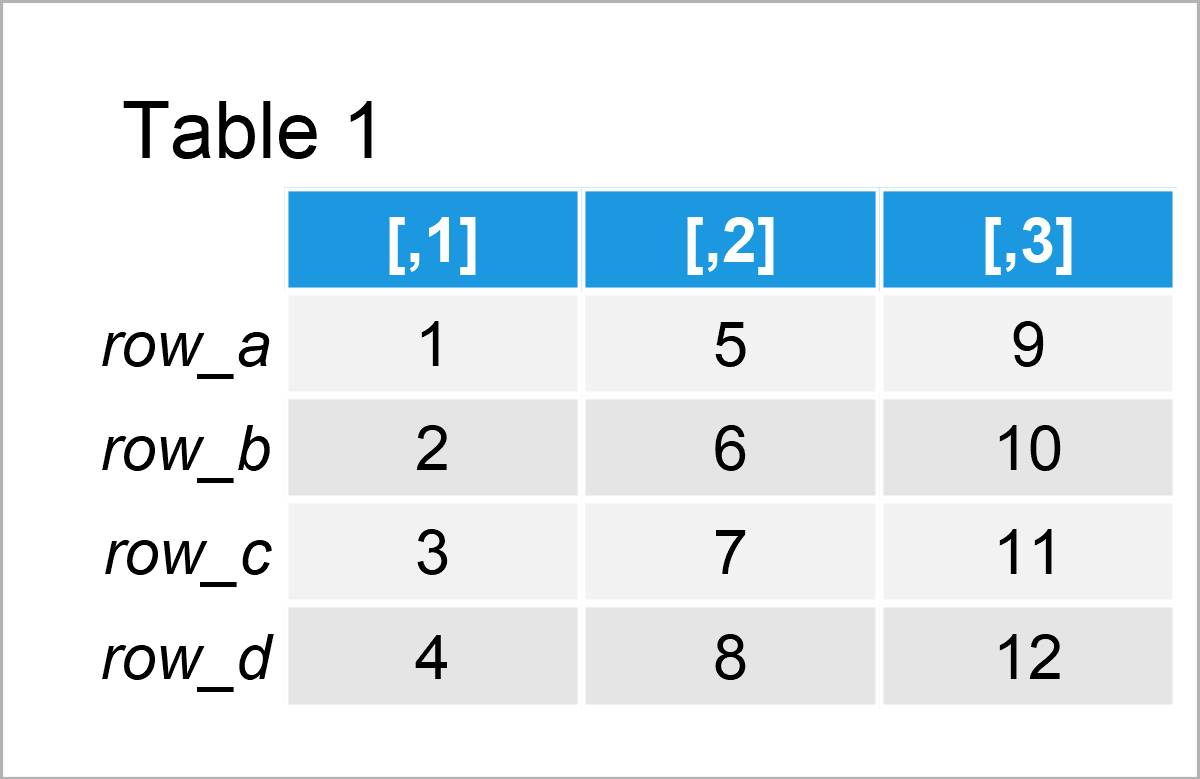# row() Function in R (2 Examples) | Get Matrix of Row Indices or Factor Labels

This tutorial demonstrates how to return row indices and labels using the row() function in the R programming language.

The article consists of these topics:

Let’s take a look at some R codes in action.

## Construction of Example Data

At first, let’s construct some example data in R:

```my_mat <- matrix(1:12, nrow = 4)    # Create example matrix
row.names(my_mat) <- paste0("row_", letters[1:4])
my_mat                              # Print example matrix```Have a look at the previous table. It shows that our example data matrix is composed of four rows and the three integer columns.

## Example 1: Apply row() Function to Return Matrix of Integers Indicating Row Numbers

This example shows how to return a matrix of row numbers using the row function.

Have a look at the following R code:

`row(my_mat)                         # Apply row function`The output of the previously shown R code is shown in Table 2 – A matrix object that contains the row numbers for each column of our input matrix.

## Example 2: Apply row() Function to Return Matrix of Row Labels

This example shows how to get the row labels (i.e. the row names) of a matrix object using the row function.

For this, we have to set the as.factor argument within the row function to be equal to TRUE:

```row(my_mat, as.factor = TRUE)       # Apply row function
#      [,1]  [,2]  [,3]
# [1,] row_a row_a row_a
# [2,] row_b row_b row_b
# [3,] row_c row_c row_c
# [4,] row_d row_d row_d
# Levels: row_a row_b row_c row_d```

The previous R code has returned a matrix-like factor object to the RStudio console that contains the row labels of each row.

## Video, Further Resources & Summary

Would you like to know more about the application of the row() function? Then you may have a look at the following video on my YouTube channel. In the video, I explain the R programming codes of this tutorial:

In addition, you might have a look at the other articles on my website. You can find a selection of other articles below.

In this tutorial you have learned how to apply the row() function in the R programming language. In case you have additional questions or comments, tell me about it in the comments below.

Subscribe to the Statistics Globe Newsletter